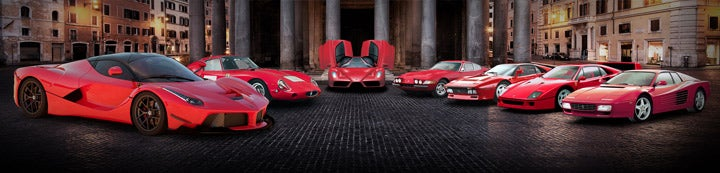1 - 1 of 1 Posts

#### Angelis

·
##### Registered
Joined
·
603 Posts
Discussion Starter · ·
Some figures I have for Ferrari's produced. Got it from a booklet I picked up.

FERRARI Production numbers

Model - Total - (RHD)

308 = 12,143 (965)
328 = 7,412 (672)
348 = 8,654 (496)
355 = 11,206 (1,384)
360 = 17,800

308

1976 - 308 GTB (Fibre glass) = 712 - RHD UK = 154
1976 - 328 GTS (steel) = 2,185 - RHD UK = 211
1978 - 308 GTS = 3,219 - RHD UK = 184
1981 - 308 GTBi = 494 - RHD UK = 42
1981 - 308 GTSi = 1,743 - RHD UK = 67
1982 - 308 GTB QV = 748 - RHD UK = 74
1981 - 308 GTS QV = 3,042 - RHD UK = 233

Total = 12,143

UK RHD = 965

328

328 GTB = 1,344 - RHD UK = 130 (53 ABS)
328 GTS = 6068 - RHD UK = 542 (292 ABS)

Total = 7,412

UK RHD = 672

348

348tb = 2,895 - RHD UK = 130
348ts = 4,230 - RHD UK = 261
348spider = 1090 - RHD UK = 68
348GTB = 252 - RHD UK = 14
348GTS = 137 - RHD UK = 15
348GTC = 50 - RHD UK = 8

Total = 8,654

UK RHD = 496

355

355 B= 4915 - RHD UK = 496
355 GTS= 2577 - RHD UK = 434
355 Spider= 3714 - RHD UK = 454

Total = 11,206

UK RHD = 1,384

360

360 Modena - 8,800
360 Spider - 7,565
Challenge Stradale - 1,274
360 Challenge - 200

Total = 17,800

1 - 1 of 1 Posts